# Class 8 Maths NCERT Solutions for Chapter – 2 Linear Equations In One Variable Ex – 2.5

### Linear Equations In One Variable

Question 1.$\frac { x }{ 2 } -\frac { 1 }{ 5 } =\frac { x }{ 3 } +\frac { 1 }{ 4 }$
Solution: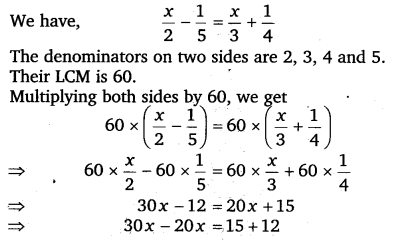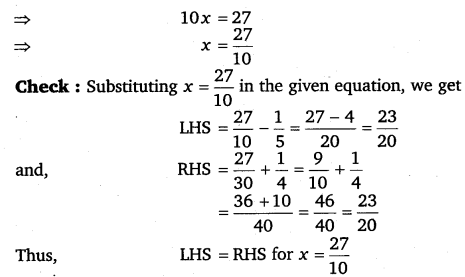Question 2.$\frac { n }{ 2 } -\frac { 3n }{ 4 } +\frac { 5n }{ 6 } =21$
Solution: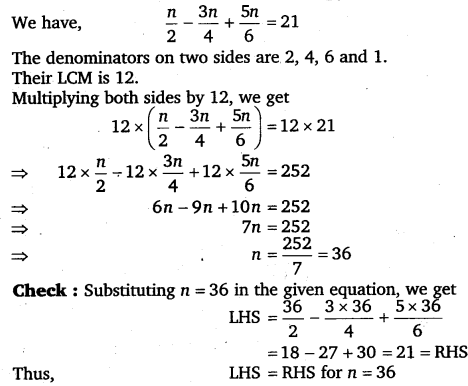Question 3.$x+7-\frac { 8x }{ 3 } =\frac { 17 }{ 6 } -\frac { 5x }{ 2 }$
Solution: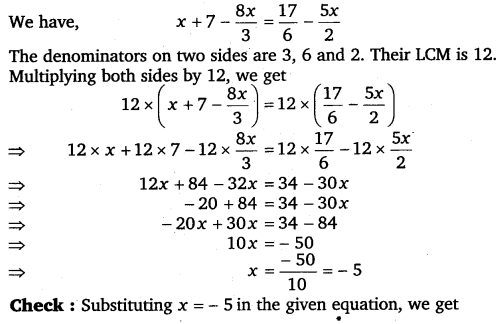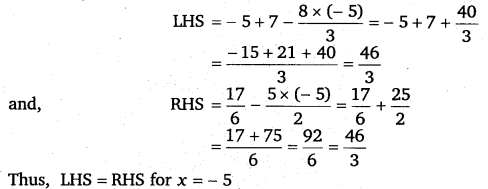Question 4.$\frac { x-5 }{ 3 } =\frac { x-3 }{ 5 }$
Solution: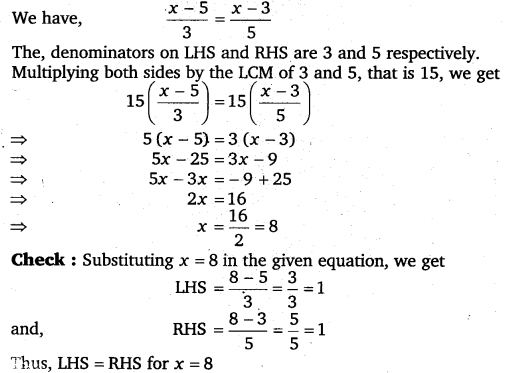Question 5.$\frac { 3t-2 }{ 4 } -\frac { 2t+3 }{ 3 } =\frac { 2 }{ 3 } -t$
Solution: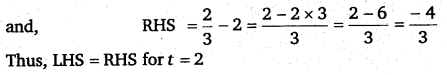Question 6.$m-\frac { m-1 }{ 2 } =1-\frac { m-2 }{ 3 }$
Solution:Simplify and solve the following linear equations:

Question 7.
3(t – 3) = 5(2t + 1)

Solution:Question 8.
15(y – 4) – 2 (y – 9) + 5(y + 6) = 0

Solution: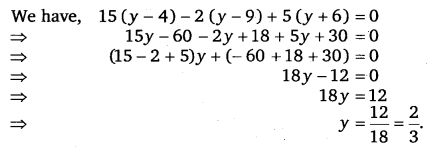Question 9.
3(5z – 7) – 2(9z – 11) = 4(8z – 13) – 17

Solution:Question 10.
0.25(4f – 3) = 0.05(10f – 9).

Solution: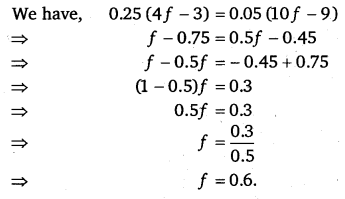### Take Your IIT JEE Coaching to Next Level with Examtube

• Mentoring & Teaching by IITians
• Regular Testing & Analysis
• Preparation for Various Engineering Entrance Exams
• Support for School/Board Exams